# Chapter 7 s Class 2 BreakEven Analysis u

• Slides: 31Chapter 7 s Class 2Break-Even Analysis u Technique for evaluating process and equipment alternatives u Objective is to find the point in dollars and units at which cost equals revenue u Requires estimation of fixed costs, variable costs, and revenue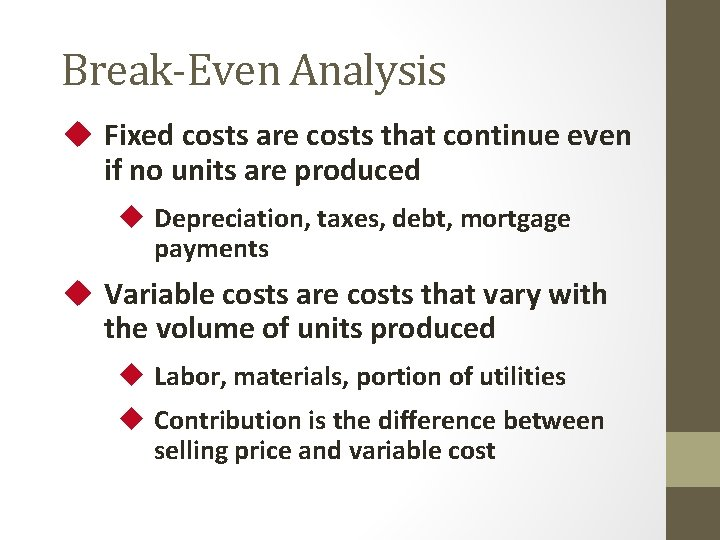Break-Even Analysis u Fixed costs are costs that continue even if no units are produced u Depreciation, taxes, debt, mortgage payments u Variable costs are costs that vary with the volume of units produced u Labor, materials, portion of utilities u Contribution is the difference between selling price and variable costBreak-Even Analysis – Total revenue line 900 – 800 – Break-even point Total cost = Total revenue 700 – Cost in dollars r o it c f o r P 600 – do rri Total cost line 500 – Variable cost 400 – 300 – 200 – 100 – – 0 | Figure S 7. 5 ss r Lo ido rr co | | Fixed cost | | | | | 100 200 300 400 500 600 700 800 900 1000 1100 Volume (units period)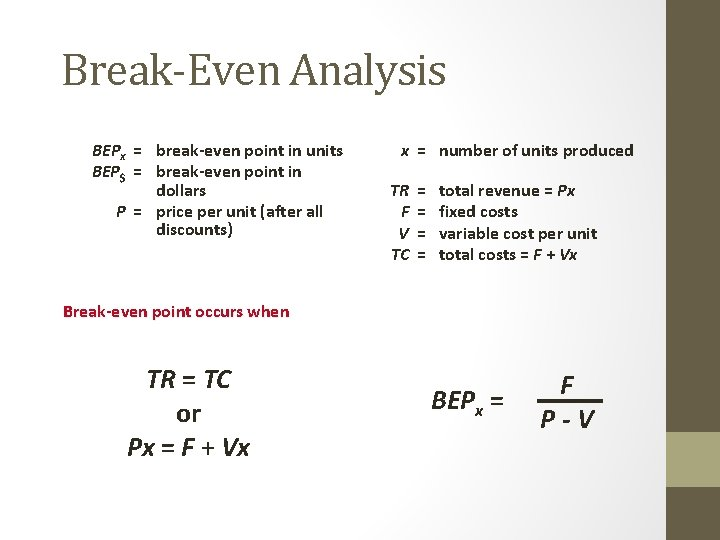Break-Even Analysis BEPx = break-even point in units BEP\$ = break-even point in dollars P = price per unit (after all discounts) x = number of units produced TR F V TC = = total revenue = Px fixed costs variable cost per unit total costs = F + Vx Break-even point occurs when TR = TC or Px = F + Vx BEPx = F P-V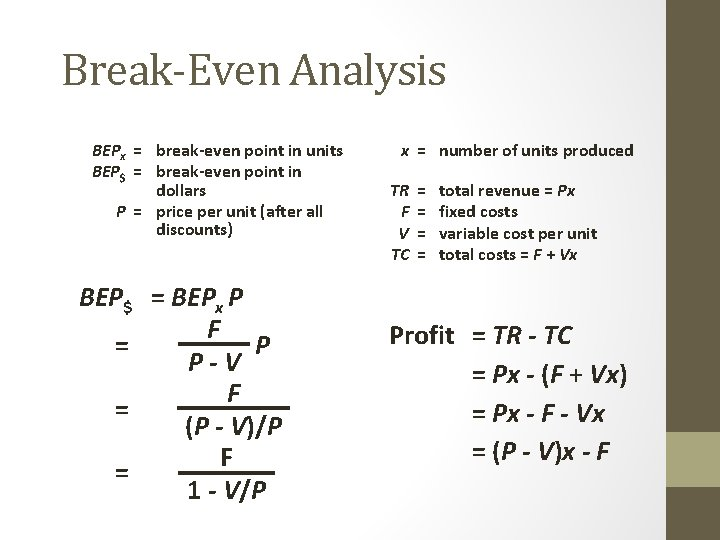Break-Even Analysis BEPx = break-even point in units BEP\$ = break-even point in dollars P = price per unit (after all discounts) BEP\$ = BEPx P F = P P-V F = (P - V)/P F = 1 - V/P x = number of units produced TR F V TC = = total revenue = Px fixed costs variable cost per unit total costs = F + Vx Profit = TR - TC = Px - (F + Vx) = Px - F - Vx = (P - V)x - F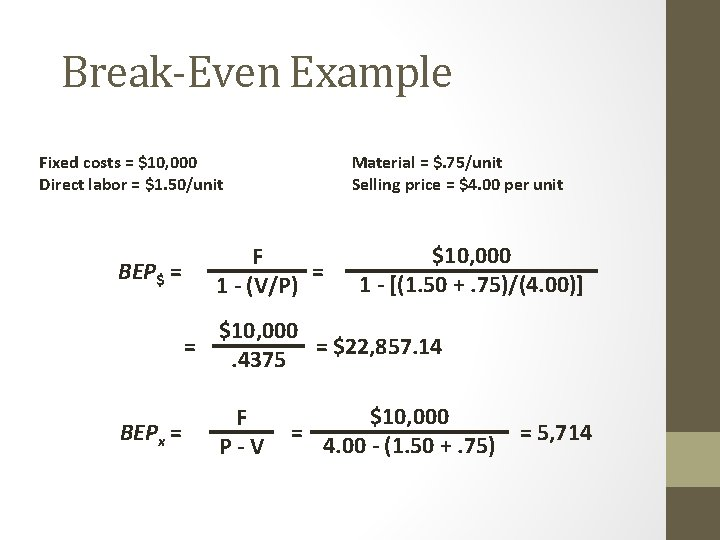Break-Even Example Fixed costs = \$10, 000 Direct labor = \$1. 50/unit BEP\$ = Material = \$. 75/unit Selling price = \$4. 00 per unit F = 1 - (V/P) \$10, 000 1 - [(1. 50 +. 75)/(4. 00)] \$10, 000 = = \$22, 857. 14. 4375 BEPx = F P-V \$10, 000 = 4. 00 - (1. 50 +. 75) = 5, 714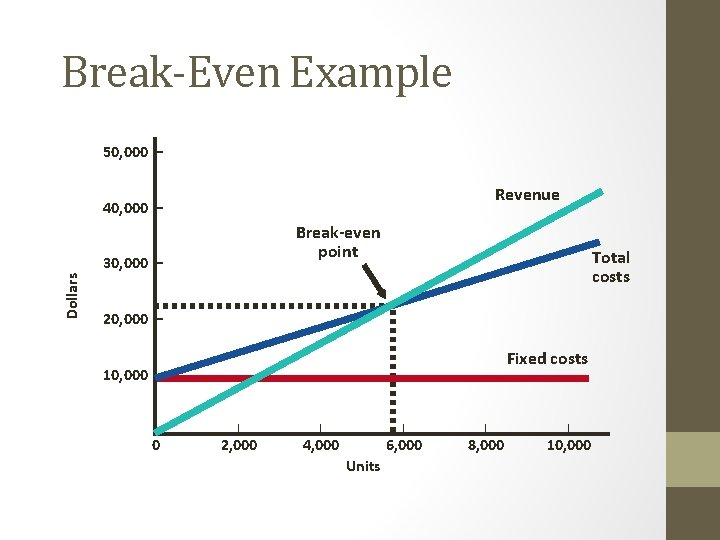Break-Even Example 50, 000 – Revenue 40, 000 – Break-even point Dollars 30, 000 – Total costs 20, 000 – Fixed costs 10, 000 – |– 0 | 2, 000 | | 4, 000 6, 000 Units | 8, 000 | 10, 000Problem S 7. 23 An electronic firm is currently manufacturing an item that has a variable cost of \$0. 5 per unit and a selling price of \$1. 00 per unit. Fixed costs are \$14, 000. Current volume is 30, 000 units. The firm can substantially improve the product quality by adding a new piece of equipment at an additional fixed cost of \$6, 000. Variable cost would increase to \$0. 60, but volume should jump to 50, 000 units due to a higher quality product. Should the company buy the new equipment?Problem S 7. 23 • Option A: Stay as is • Option B: add new equipment Therefore, the company should stay with the current equipment.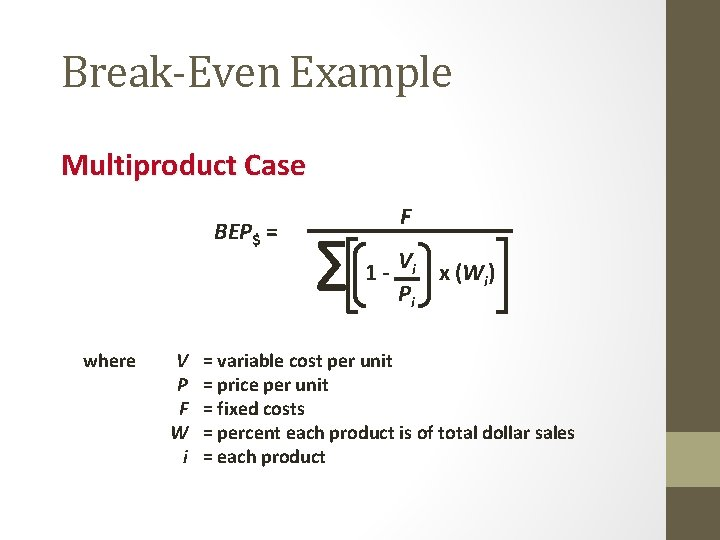Break-Even Example Multiproduct Case BEP\$ = where V P F W i ∑ F Vi 1 x (Wi) Pi = variable cost per unit = price per unit = fixed costs = percent each product is of total dollar sales = each product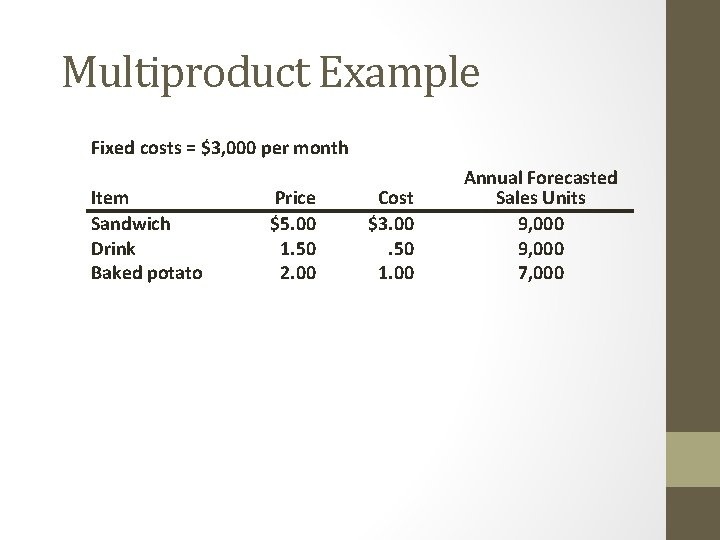Multiproduct Example Fixed costs = \$3, 000 per month Item Sandwich Drink Baked potato Price \$5. 00 1. 50 2. 00 Cost \$3. 00. 50 1. 00 Annual Forecasted Sales Units 9, 000 7, 000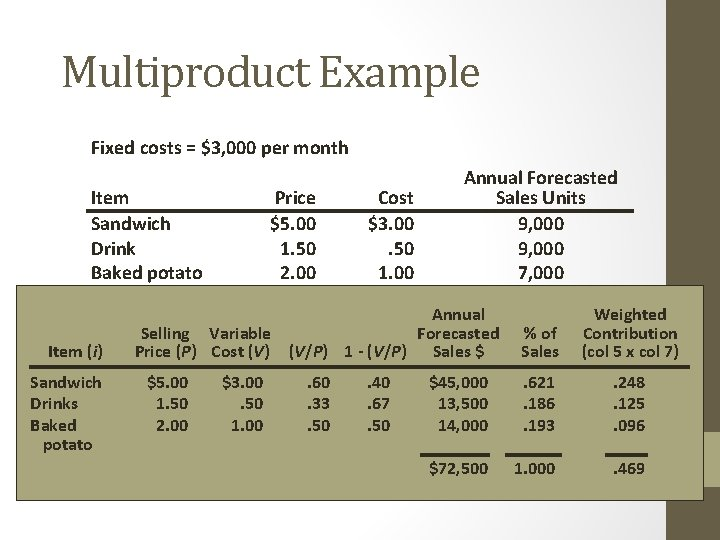Multiproduct Example Fixed costs = \$3, 000 per month Item Sandwich Drink Baked potato Item (i) Sandwich Drinks Baked potato Price \$5. 00 1. 50 2. 00 Cost \$3. 00. 50 1. 00 Annual Forecasted Sales Units 9, 000 7, 000 Annual Selling Variable Forecasted Price (P) Cost (V) (V/P) 1 - (V/P) Sales \$ \$5. 00 1. 50 2. 00 \$3. 00. 50 1. 00 . 60. 33. 50 . 40. 67. 50 % of Sales Weighted Contribution (col 5 x col 7) \$45, 000 13, 500 14, 000 . 621. 186. 193 . 248. 125. 096 \$72, 500 1. 000 . 469F Multiproduct Example BEP = V ∑ 1 - P \$ i Fixed costs = \$3, 000 per month Item Sandwich Drink Baked potato Item (i) Sandwich Drinks Baked potato Price \$5. 00 1. 50 2. 00 Selling Variable Price (P) Cost (V) (V/P) \$5. 00 1. 50 2. 00 \$3. 00. 50 1. 00 . 60. 33. 50 i x (Wi) Annual Forecasted \$3, 000 x 12 = \$76, 759 Cost = Sales Units. 469 \$3. 00 9, 000. 50 9, 000 \$76, 759 Daily 1. 00 7, 000= \$246. 02 sales = 312 days Annual Weighted Forecasted. 621 x \$246. 02 % of Contribution = 30. 6(col 31 1 - (V/P) Sales 5 x col 7) \$5. 00\$ sandwiches. 40 \$45, 000. 621 per day. 248. 67 13, 500. 186. 125. 50 14, 000. 193. 096 \$72, 500 1. 000 . 469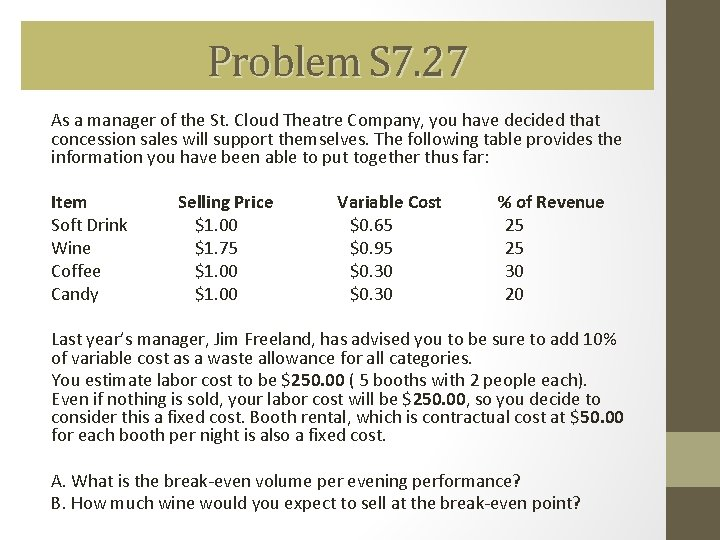Problem S 7. 27 As a manager of the St. Cloud Theatre Company, you have decided that concession sales will support themselves. The following table provides the information you have been able to put together thus far: Item Soft Drink Wine Coffee Candy Selling Price Variable Cost % of Revenue \$1. 00 \$0. 65 25 \$1. 75 \$0. 95 25 \$1. 00 \$0. 30 30 \$1. 00 \$0. 30 20 Last year’s manager, Jim Freeland, has advised you to be sure to add 10% of variable cost as a waste allowance for all categories. You estimate labor cost to be \$250. 00 ( 5 booths with 2 people each). Even if nothing is sold, your labor cost will be \$250. 00, so you decide to consider this a fixed cost. Booth rental, which is contractual cost at \$50. 00 for each booth per night is also a fixed cost. A. What is the break-even volume per evening performance? B. How much wine would you expect to sell at the break-even point?Problem S 7. 27 (a) total fixed cost = labor (\$250) + booth rental (5 \$50) = \$500. BEP\$ = ∑ F 1 - Vi x (Wi) Pi = 500 0. 507 = \$76, 759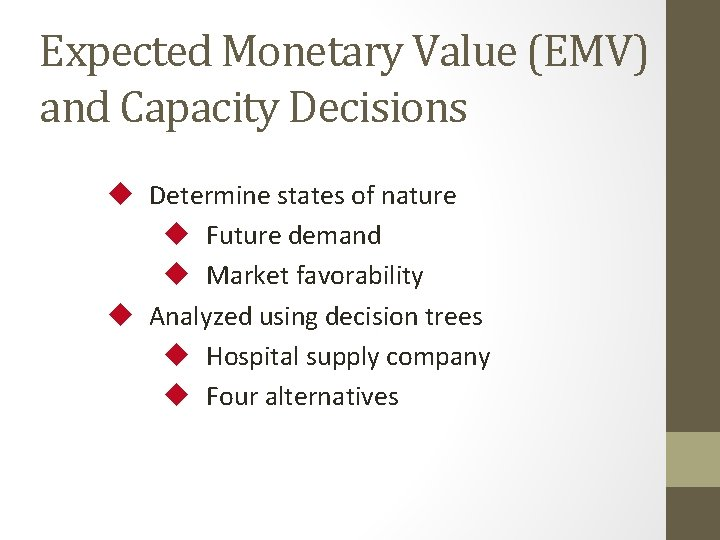Expected Monetary Value (EMV) and Capacity Decisions u Determine states of nature u Future demand u Market favorability u Analyzed using decision trees u Hospital supply company u Four alternatives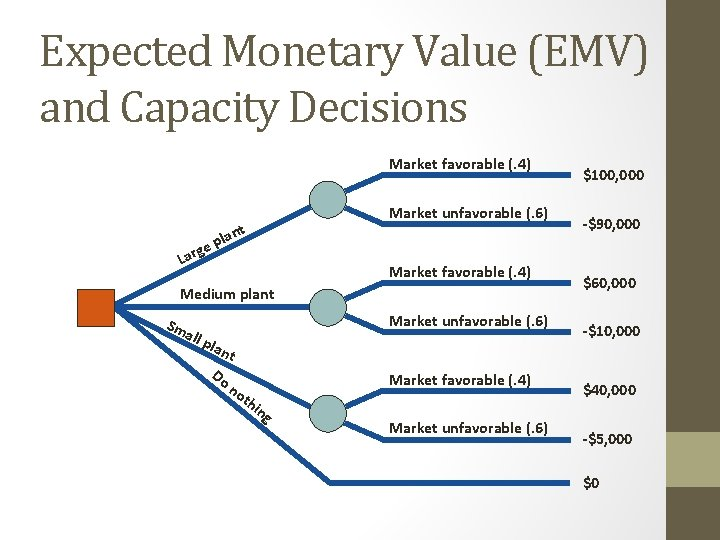Expected Monetary Value (EMV) and Capacity Decisions Market favorable (. 4) Market unfavorable (. 6) t lan p e g Lar Market favorable (. 4) Medium plant Sm all Market unfavorable (. 6) pla n \$100, 000 -\$90, 000 \$60, 000 -\$10, 000 t Do n Market favorable (. 4) ot hin g Market unfavorable (. 6) \$40, 000 -\$5, 000 \$0Expected Monetary Value (EMV) and Capacity Decisions Market favorable (. 4) Market unfavorable (. 6) t lan p e g Lar Market favorable (. 4) Medium plant Sm. Large Plant all pla nt Market unfavorable (. 6) EMV = Do (. 4)(\$100, 000) no th + (. 6)(-\$90, 000) in Market favorable (. 4) EMV = -\$14, 000 Market unfavorable (. 6) g \$100, 000 -\$90, 000 \$60, 000 -\$10, 000 \$40, 000 -\$5, 000 \$0Expected Monetary Value (EMV) and Capacity Decisions -\$14, 000 Market favorable (. 4) Market unfavorable (. 6) t lan p e g Lar all -\$90, 000 \$18, 000 Market favorable (. 4) Medium plant Sm \$100, 000 Market unfavorable (. 6) pla n t \$60, 000 -\$10, 000 \$13, 000 Do n Market favorable (. 4) ot hin g Market unfavorable (. 6) \$40, 000 -\$5, 000 \$0Problem S 7. 28 James Lawson's Bed and Breakfast, in a small historic Mississippi town, must decide how to subdivide (remodel) the large old home that will become its inn. There are three alternatives: Option A would modernize all baths and combine rooms, leaving the inn with four suites, each suitable for two to four adults. Option B would modernize only the second floor; the results would be six suites, four for two to four adults, two for two adults only. Option C (the status quo option) leaves all walls intact. In this case, there are eight rooms available, but only two are suitable for four adults, and four rooms will not have private baths. Below are the details of profit and demand patterns that will accompany each option: Alternatives A (modernize all) B (modernize 2 nd) C (status quo) High P \$90, 000 0. 5 \$80, 000 0. 4 \$60, 000 0. 3 Average P 25, 000 0. 5 \$70, 000 0. 6 \$55, 000 0. 7 Which option has the highest expected monetary value EMV?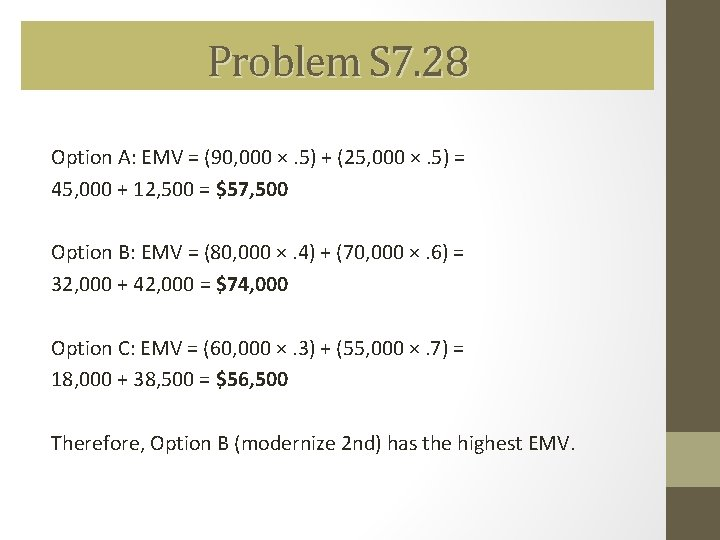Problem S 7. 28 Option A: EMV = (90, 000 ×. 5) + (25, 000 ×. 5) = 45, 000 + 12, 500 = \$57, 500 Option B: EMV = (80, 000 ×. 4) + (70, 000 ×. 6) = 32, 000 + 42, 000 = \$74, 000 Option C: EMV = (60, 000 ×. 3) + (55, 000 ×. 7) = 18, 000 + 38, 500 = \$56, 500 Therefore, Option B (modernize 2 nd) has the highest EMV.Problem S 7. 28 Decision tree solution: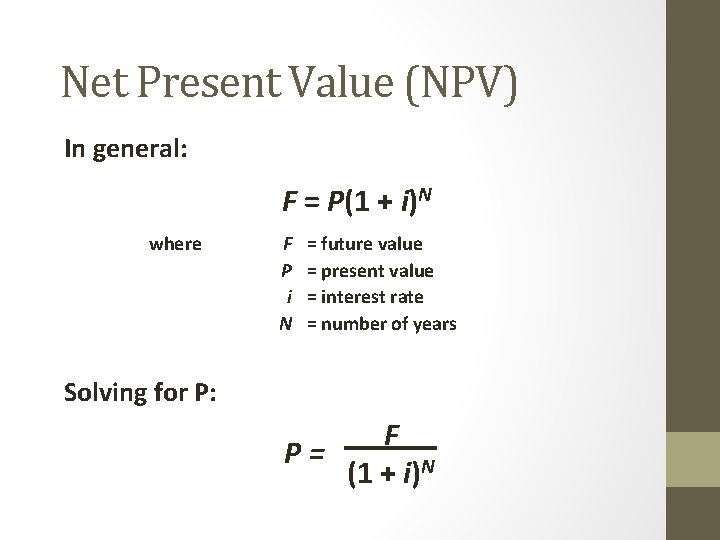Net Present Value (NPV) In general: F = P(1 + i)N where F P i N = future value = present value = interest rate = number of years Solving for P: F P= (1 + i)NNet Present Value (NPV) In general: F = P(1 + i)N where F P i N = future value = present value this works fine, While = interest rate is cumbersome for = number of years larger values of N Solving for P: F P= (1 + i)N it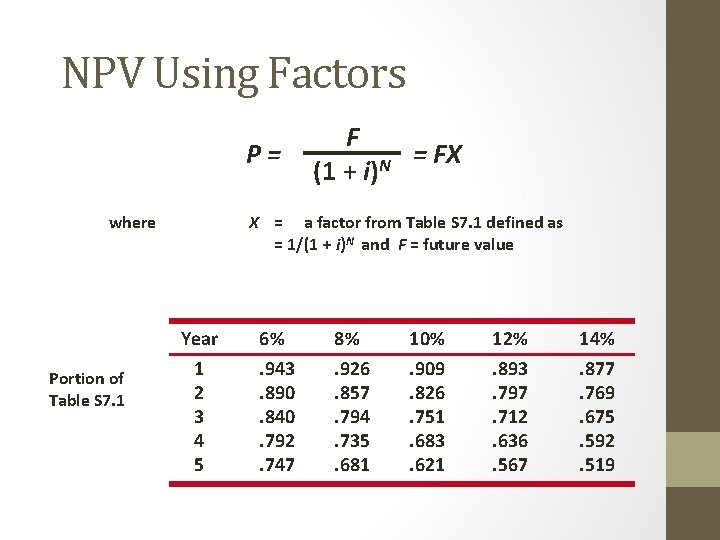NPV Using Factors P= where Portion of Table S 7. 1 F = FX N (1 + i) X = a factor from Table S 7. 1 defined as = 1/(1 + i)N and F = future value Year 6% 8% 10% 12% 14% 1 2 3 4 5 . 943. 890. 840. 792. 747 . 926. 857. 794. 735. 681 . 909. 826. 751. 683. 621 . 893. 797. 712. 636. 567 . 877. 769. 675. 592. 519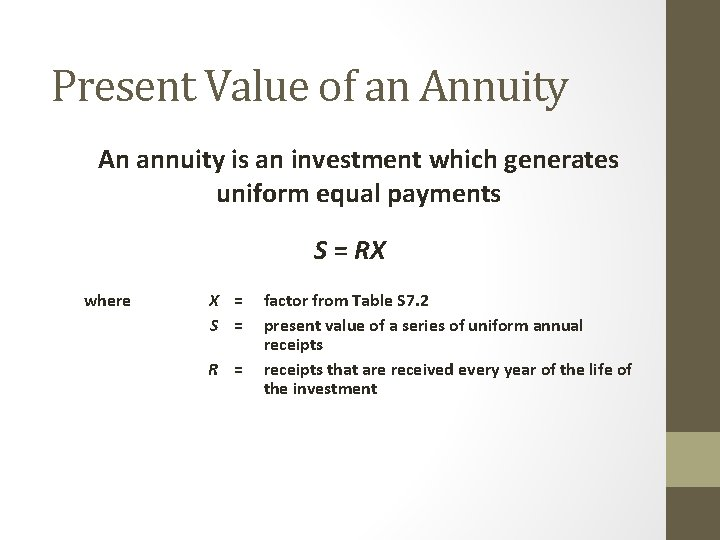Present Value of an Annuity An annuity is an investment which generates uniform equal payments S = RX where X = S = R = factor from Table S 7. 2 present value of a series of uniform annual receipts that are received every year of the life of the investment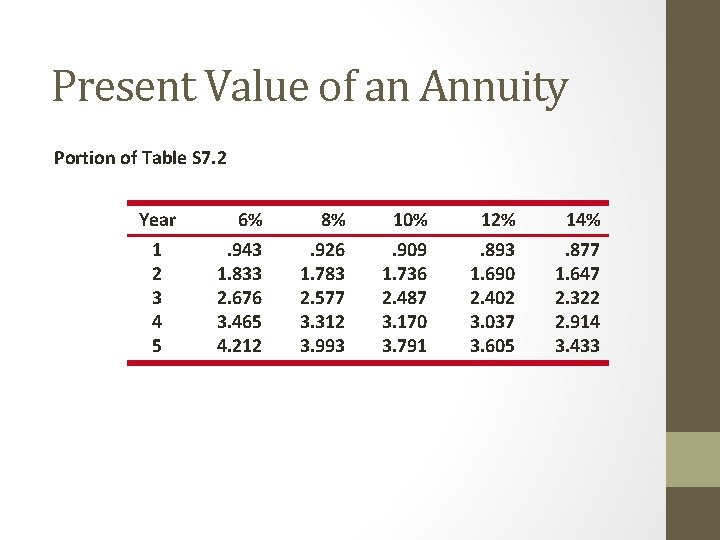Present Value of an Annuity Portion of Table S 7. 2 Year 1 2 3 4 5 6% 8% 10% 12% 14% . 943 1. 833 2. 676 3. 465 4. 212 . 926 1. 783 2. 577 3. 312 3. 993 . 909 1. 736 2. 487 3. 170 3. 791 . 893 1. 690 2. 402 3. 037 3. 605 . 877 1. 647 2. 322 2. 914 3. 433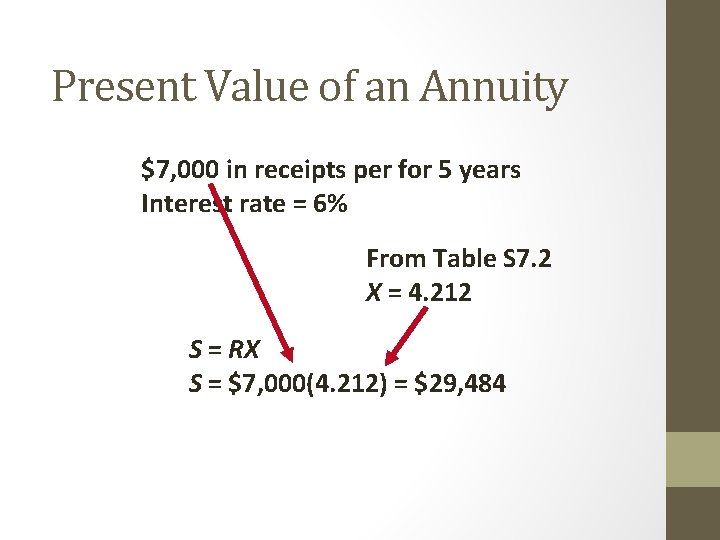Present Value of an Annuity \$7, 000 in receipts per for 5 years Interest rate = 6% From Table S 7. 2 X = 4. 212 S = RX S = \$7, 000(4. 212) = \$29, 484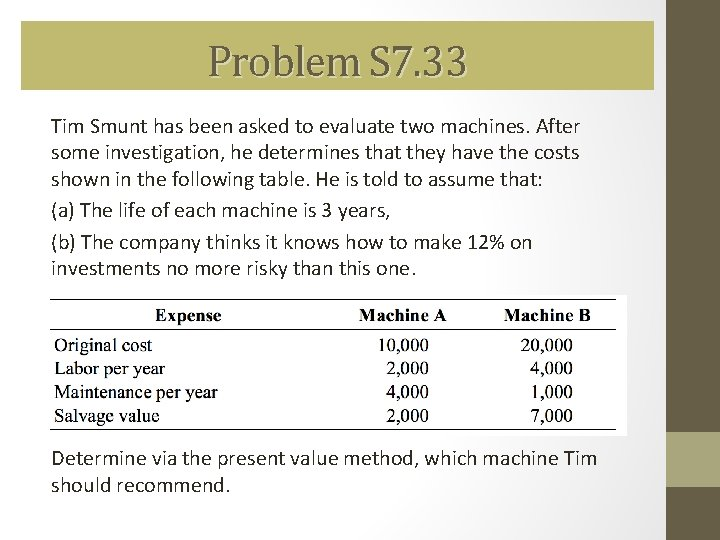Problem S 7. 33 Tim Smunt has been asked to evaluate two machines. After some investigation, he determines that they have the costs shown in the following table. He is told to assume that: (a) The life of each machine is 3 years, (b) The company thinks it knows how to make 12% on investments no more risky than this one. Determine via the present value method, which machine Tim should recommend.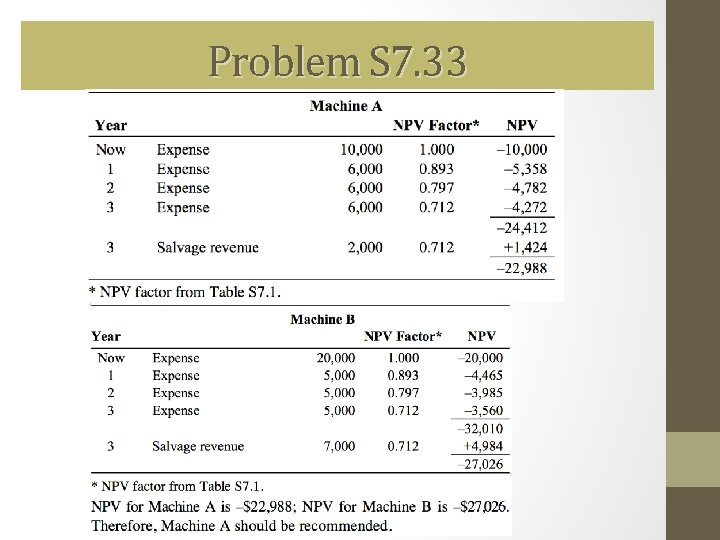Problem S 7. 33